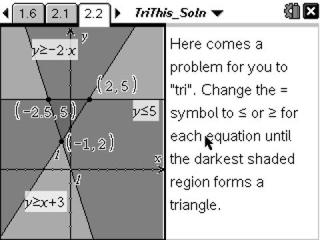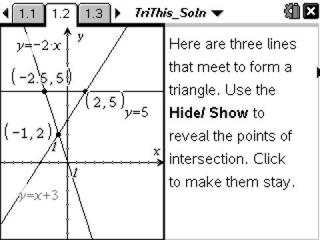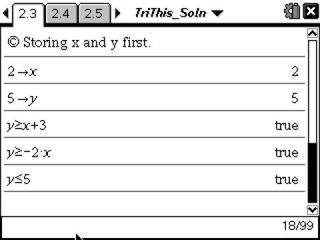# Activities

••• ##### Subject Area

• Math: Algebra I: Linear Functions

• ##### Author9-12

30 Minutes

• ##### Device
• TI-Nspire™ Navigator™
• TI-Nspire™
• TI-Nspire™ CAS
• ##### Software

TI-Nspire™
TI-Nspire™ CAS

2.0

• ##### Report an Issue

Tri This!#### Activity Overview

Students investigate linear equations that form a triangle and determine which vertex is a solution to a system of equations.

#### Key Steps

•In Problem 1, students will see three intersecting lines that form a triangle. After revealing the coordinates of the intersection points, students will determine the systems of two linear equations whose solutions describe the points. They will also determine if a point inside the triangle is a solution to any of the systems.

•In Problem 2, students will change the equations given in the first problem to inequalities, where the darkest shaded region forms the triangle.

•Students will use a Calculator page to determine if the vertices and points inside the triangle are solutions to the system of three linear inequalities. Students will conclude the activity by describing any differences they found in solutions between the systems of equations and the system of inequalities.